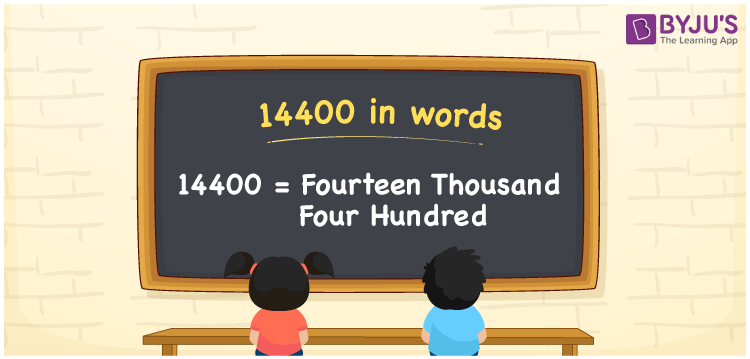# 14400 in Words

We can express 14400 in words as Fourteen Thousand Four Hundred. 14400 can be written in words using the ones, tens, hundreds, thousands place of a number. We know that 14400 is a cardinal number or counting number as it depicts a specific quantity. Place value charts are important to write any number in words. Let us learn how to write the number name of 14400 in this article.

 14400 in words Fourteen Thousand Four Hundred Fourteen Thousand Four Hundred in numerical form 14400

## 14400 in English Words

Generally, numbers in words are written using the English alphabet. Therefore, English is vital in learning how to write and read numbers in words. Hence, 14400 in words is written as Fourteen Thousand Four Hundred.## How to Write 14400 in Words?

To write numbers in words, first, identify the place value of each digit in the given number with the help of a place value chart. The place value chart for the number 14400 is given below.

 Ten-Thousands Thousands Hundreds Tens Ones 1 4 4 0 0

Therefore, we can write the expanded form as:

1 x Ten Thousand + 4 x Thousand + 4 x Hundred + 0 × Ten + 0 × One

= 1 x 10000 + 4 x 1000 + 4 x 100 + 0 + 0

= 10000 + 4000 + 400

= 14400

= Fourteen Thousand Four Hundred

Hence, 14400 in words is written as Fourteen Thousand Four Hundred.

Interesting way of writing 14400 in words

1 = One

14 = Fourteen

144 = One Hundred and Forty-Four

1440 = One Thousand Four Forty

14400 = Fourteen Thousand Four Hundred

Therefore, the word form of the number 14400 is Fourteen Thousand Four Hundred.

14400 is a natural number that is succeeded by 14399 and preceded by 14401

• 14400 in words – Fourteen Thousand Four Hundred
• Is 14400 an odd number? – No
• Is 14400 an even number? – Yes
• Is 14400 a perfect square number? – Yes
• Is 14400 a perfect cube number? – No
• Is 14400 a prime number? – No
• Is 14400 a composite number? – Yes

## Frequently Asked Questions on 14400 in Words

Q1

### Write 14400 in words.

14400 in words is Fourteen Thousand Four Hundred.
Q2

### Express the value 6200 plus 8200 and write the answer in words.

6200 + 8200 = 14400 The required answer 14400 in words is written as Fourteen Thousand Four Hundred.
Q3

### How to write Fourteen Thousand Four Hundred in numbers?

Fourteen Thousand Four Hundred in numbers is 14400.